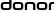## Idea: Image Contribution Probability Optimized Path Generation

Discussion related to the Engine functionality, implementations and API.
FarbigeWeltPosts: 920
Joined: Sun Jul 01, 2018 12:07 pm
Location: Switzerland
Contact:

### Idea: Image Contribution Probability Optimized Path Generation

Idea: Image Contribution Probability Optimized Path Generation
Scene
Imagine a room with an open window. Daylight from sun and sky. Room‘s window is located in the shadow. There is an upstream light lamp on the ceiling and a desktop spot light. On the desk lies an organic LED display showing the logo of LuxCoreRender, a folded newspaper of Today‘s CGI World, its upper half of the front page on top partly in the spot light beam and a square lined notebook with a thick ultramarine ball pen. The camera view includes parts of the wooden desk, main aspect is the view through a lens showing zoomed pixels of colorful shining pixels of a display breadboard.
Searched
Increased render efficiency
Background
E.g. a point lamp emits rays in every space angle but often only parts of them reach camera view after depth number of bounces. Even if all paths connect to camera lots of them lost most of their energy and their contribution to final render is negligible.
Light issues of described scene
There are:
a) Two powerful lights a1) sun and a2) sky. Light from sky enters room directly, sun in best case indirectly.
b) An indirect ceiling lamp
c) A close to camera spot light
d) Lots of tiny but enlarged weak area lights
Brightness (=probability of emitting rays) a1>a2>>b=c>>d;
First improvement:
estimated -> 1e6>1e5>>1>>1e-3; logarithmic -> 6>5>1>-3; ‚normalized‘ -> 9>8>4>1; i.e. sun emits in this case only 9 times the rays of one LED.
Second improvement:
Triangle tesselation of light‘s surface area, point light treated as outer surface of a tiny sphere, sun treated as one side of a circle area, sky as huge inner surface of a sphere; tesselation triangles‘ size depending on light area, their number is equal and default 60. Each triangle (if not from distant star or laser) emits homogeneous light in half of space angles.
Third improvement:
Because an object gets smaller the flatter its viewed angle is, light distribution can follow this fact, i.e. probability to emit a ray increases from phi=0 degree (ray in plane of triangle surface) to 90 degree (ray rectangular to triangle surface), simplified as p=sin(phi). First level possible emit angles per triangle are 180*360 = 64’800, i.e. 60*64’800 = 3’888 k angles per light source, multiplied by its logarithmic and normalized importance this means for sun 9*3’888 k = 34.992 M angles.
Forth improvement:
1 ) Contribution to render image of each ray’s source condition (light, center location tesselation triangle, space angles) gets tracked. After first pass, e.g. all lights emitted in every direction for small number of lights or for large number of lights after a fixed number of traced rays, e.g. the number of rays for a light with importance 1 and all rays with probability 1.
2 ) Before second pass rays’ probability is calculated by multiplication of contribution (0 to 1; 1 means full emitted energy) and base angle probability (sin(phi)). If probability sum of a light triangle is below 1e-3 this triangle is disabled.
3 ) All left triangles are split into six sub triangles with each‘s center as source location. Every sub triangle emits rays in every above mentioned space angle.
4 ) Passes are evaluated according to 2 and 3 until probability sum in 2) falls below a certain threshold (e.g. average probability<half of max. probability) then space angles‘ resolution is increased, i.e. 180*360*4. This resolution is also increased if in 3) number of sub triangles falls below 1/8 of number of triangles of first pass.
5 ) Steps 2), 3), 4) are repeated until halt conditions are reached.

Further improvement:
Image is split into areas of 100*100 pixels. Convergence level of each area is calculated after a number of passes. 3 sequential levels are used to estimate number of passes reaching convergence of 1-1e-3. Parabel estimation for next pass is used to
calculate probability of next pass reaching convergence of 1-1e-3. This probability is also used for the multiplication in fourth improvement 2) but for evaluation of probability sum limit is different, namely 1e-4. Probability of image areas already converged set probability to 0.
If all not yet converged areas lead to numbers of estimated passes exceeding 1e4 or the user’s max number of passes a warning could be displayed: This render may not converge in some areas of the camera view before n passes (=samples; n = min number of estimated passes).
Probability exists that some tesselation triangles or their sub triangles have been disabled to early. Last pass gets stored then first pass may be repeated at this point, instead of disabling triangles with low probability they get split into six sub triangles and triangles with high probability get disabled. Second pass is evaluated as usual until convergence pass probability succeeds or also gets to pass forecasts above 1e4 passes. At latter point previously stored pass and last pass are combined and rendering goes on as described until halt conditions are reached or user stopped rendering.
Light and Word designing Creator - www.farbigewelt.ch - aka quantenkristall || #luxcorerender
160.8 | 42.8 (10.7) Gfp | Windows 10 Pro, intel i7 4770K@3.5, 32 GB
2 AMD Radeon RX 5700 XT, 8 GB || Gfp = SFFT Gflops